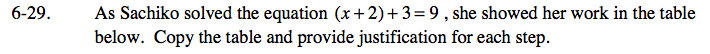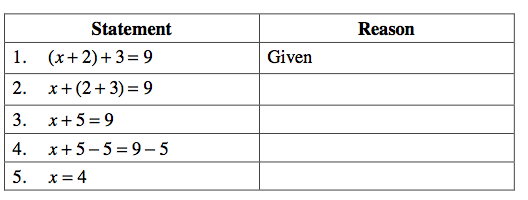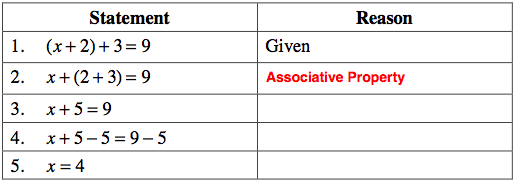### Home > CAAC > Chapter 6 > Lesson 6.1.3 > Problem6-29

6-29.

As Sachiko solved the equation (x + 2) + 3 = 9, she showed her work in the table below. Copy the table and provide justification for each step. Homework Help ✎

 Statement Reason 1. (x + 2) + 3 = 9 Given 2. x + (2 + 3) = 9 3. x + 5 = 9 4. x + 5 − 5 = 9 − 5 5. x = 4Decide what rule goes with each step being made.